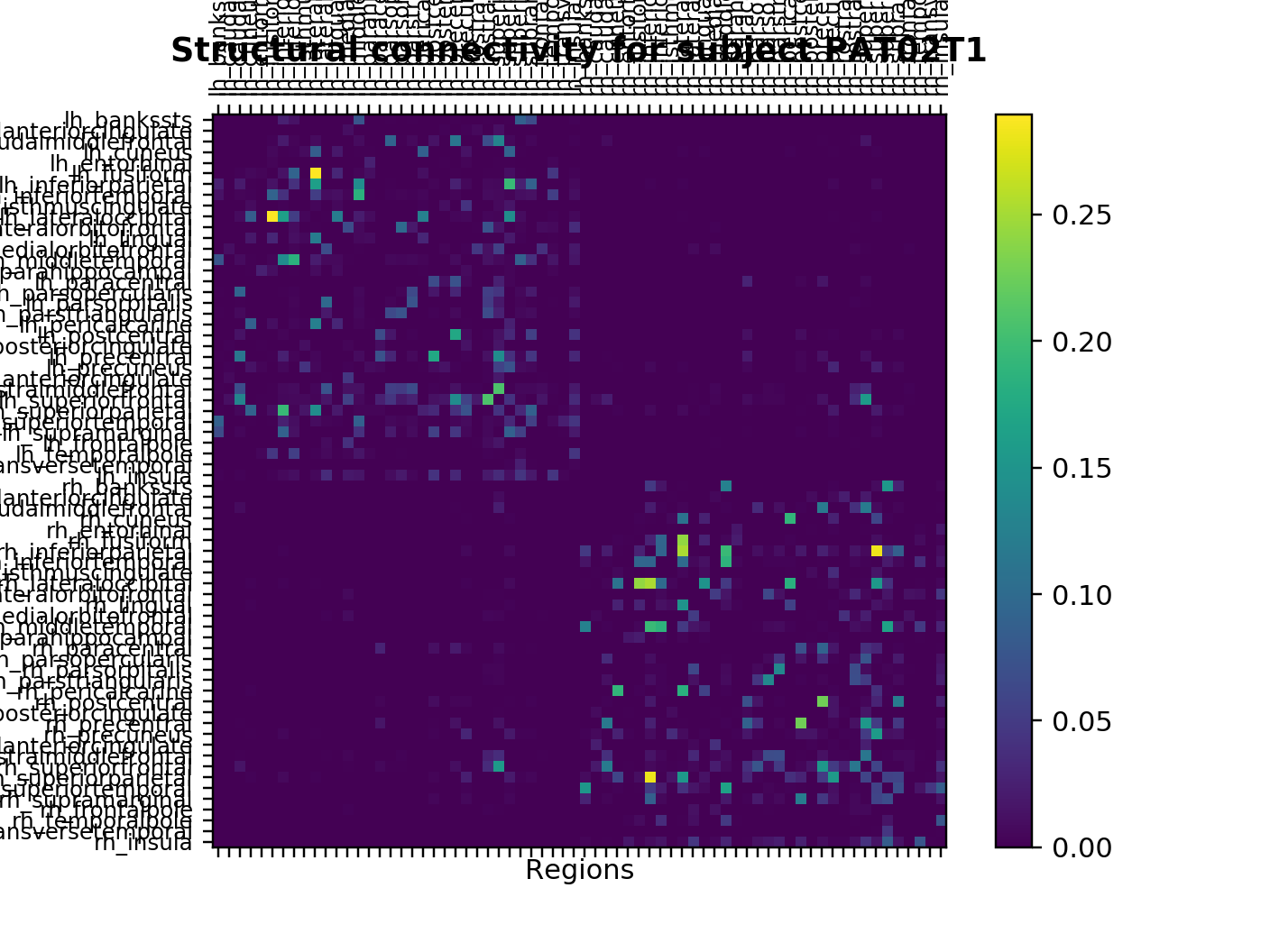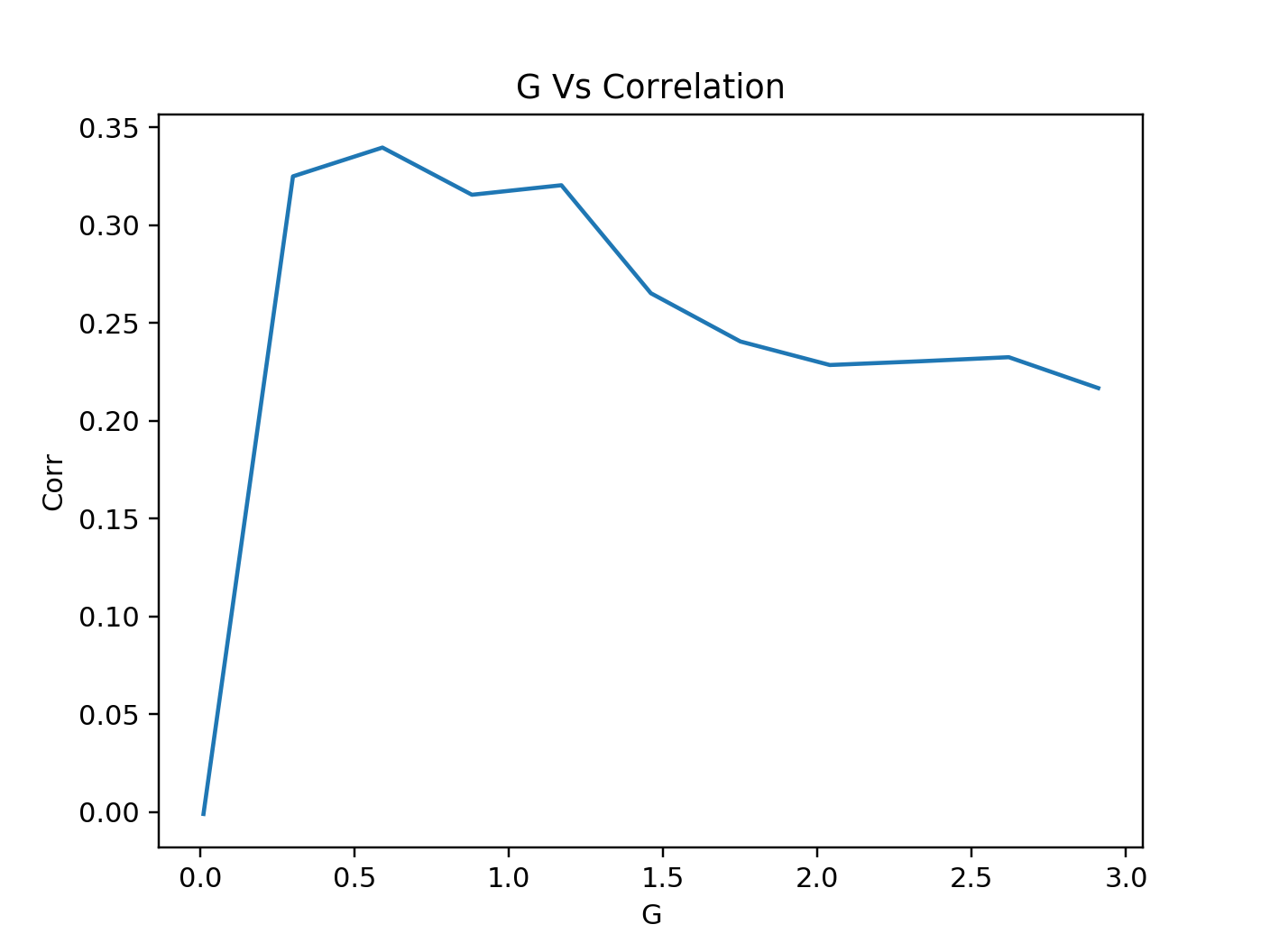# Modeling Brain Dynamics in Brain Tumor Patients Using the Virtual Brain¶

In this demo, we show how to simulate large-scale brain dynamics using The Virtual Brain, an open-source neuroinformatics platform.

In :
# load libraries
import csv
import matplotlib.pyplot as plt
import multiprocessing as mp
import numpy as np
import os
import pandas as pd
import scipy
import scipy.io as sio
import scipy.signal as sig
import scipy.stats as stat
import sys
import zipfile
from tvb.simulator.plot.tools import *
from tvb.simulator.lab import *
from tvb.simulator.models.wong_wang_exc_io_inh_i import ReducedWongWangExcIOInhI
from tvb.datatypes.time_series import TimeSeriesRegion
from tvb.analyzers import fmri_balloon
import tvb.analyzers.correlation_coefficient as corr_coeff
LOG = get_logger('BrainTumor')

In :
%pylab nbagg


## Introduction¶

We show how to simulate neural mass model with the reduced Wong-Wang model, using the individual subject connectivity. The global model parameters of the Reduced Wong-Wang model are individually optimized to improve prediction accuracy of functional connectivity.
Original publication can be found here: https://www.eneuro.org/content/5/3/ENEURO.0083-18.2018

When using The Virtual Brain for scientific publications, please cite it as follows:

Paula Sanz Leon, Stuart A. Knock, M. Marmaduke Woodman, Lia Domide,
Jochen Mersmann, Anthony R. McIntosh, Viktor Jirsa (2013)
The Virtual Brain: a simulator of primate brain network dynamics.
Frontiers in Neuroinformatics (7:10. doi: 10.3389/fninf.2013.00010)Connectivity input data can be found in the github repository: https://github.com/mlion0200/educase_TVB_braintumor, under demo_data folder.
Data contains functional and structural connectivity matrices of 25 brain tumor patients and 11 healthy control participants before surgery, and 18 brain tumor patients and 10 healthy control participants after neurosurgery.

Put Input data folder in the same directory of this python notebook. Input data needs to follow the directory structure below:

TVB_braintumor.ipynb
TVB_input
│   CONXXTX (Control/Patients + number + T1/T2)
│   │   FC.mat
│   │   SCthrAn.mat
│   └───CON02T1_TVB.zip
│       │   areas.txt
│       │   average_orientations.txt
│       │   centres.txt
│       │   cortical.txt
│       │   hemisphere.txt
│       │   tract_lengths.txt.txt
│       │   weights.txt
│
└───CONXXTX
│   ...
In :
# Set directory path
data_dir = "TVB_input"
zip_suffix = "_TVB"

In :
def load_connectivity(input_name):
zip_file_name = input_name + zip_suffix + ".zip"
dir_name = input_name + zip_suffix
zip_path = data_dir + "/" + input_name + "/" + zip_file_name
dir_path = data_dir + "/" + input_name + "/" + dir_name
conn = connectivity.Connectivity.from_file(zip_path)
# Configure, to compute derived data, such as number_of_nodes and delays
conn.configure()

# Check weight matrix from .zip is corresponding to structural connectivity matrix from matlab file.
with zipfile.ZipFile(zip_path, 'r') as zip_ref:
zip_ref.extractall(dir_path)
weight_txt = np.loadtxt(fname = dir_path + "/weights.txt")
# Load the structural connectivity matrix from matlab file
SC_path = data_dir + "/" + input_name + "/SCthrAn.mat"
assert(np.allclose(x['SCthrAn'], weight_txt), "Weight matrix in weights.txt should be the same as SCthrAn.mat")
return conn

In :
# Load the connectivity data
# Set input directory name follows "CONXXTX"
# Controls are the "CONxx", patients are "PATxx", before surgery is denoted with "T1", after surgery is "T2".
input_name = "PAT02T1"
conn.configure()

# Show connectivity summary information.
conn.summary_info

# Plot Structural Connectivity Info: A 2D plot for visualizing the Connectivity.weights matrix
plot_connectivity(connectivity = conn, plot_tracts=False)
plt.xlabel("Regions")
plt.ylabel("Regions")
connectivity_title = "Structural connectivity for subject " + input_name
plt.title(connectivity_title, fontweight="bold", fontsize="12", y = 1.05)Out:
Text(0.5,1.05,'Structural connectivity for subject PAT02T1')

## Load Empirical Functional Connectivity matrix¶

In :
# get empirical functional connectivity from FC.mat
# in this demo the functional connectivity parameter name is FC_cc_DK68, adjust to your variable name
fc_file_name = data_dir + "/" + input_name + "/FC.mat"
fc_cc_name = "FC_cc_DK68"

# indexes of all the values above the diagonal.
uidx = np.triu_indices(68, 1)

# Fisher-Z transform the correlations, important for standardization
em_fc_z = arctanh(em_fc_matrix)
# get the upper triangle since it is symmetric along diagonal
em_fc = em_fc_z[uidx]


## Configure Simulator¶

Reduced Wong-Wang model with an excitatory and an inhibitory population, mutually coupled.
Following Deco et al 2014.
Table below shows parameters defined in Reduced Wong-Wang model, default value and description.

Parameter Name Default Value Description
a_e 310 Excitatory population input gain parameter (n/C)
b_e 125 Excitatory population input shift parameter (Hz)
d_e 0.16 Excitatory population input scaling parameter (s)
gamma_e 0.641/1000 Excitatory population kinetic parameter
tau_e 100 Excitatory population NMDA decay time constant (ms)
w_p 1.4 Excitatory population recurrence weight
J_N 0.15 NMDA current (nA)
W_e 1.0 Excitatory population external input scaling weight
a_i 615 Inhibitory population input gain parameter (n/C)
b_i 177 Inhibitory population input shift parameter (Hz)
d_i 0.087 Inhibitory population input scaling parameter (s)
gamma_i 1.0/1000 Inhibitory population kinetic parameter
tau_i 10 Inhibitory population NMDA decay time constant (ms)
J_i 1.0 Local inhibitory current (nA)
I_o 0.382 Effective external input (nA)
G 2.0 Global coupling scaling (To be optimized)
lamda 0.0 Inhibitory global coupling scaling
In :
# set up the Reduced Wong-Wang model
# adjust the parameters to your needs, otherwise it will use the default value shown above
rww = ReducedWongWangExcIOInhI()

In :
# set up the simulator
# in the demo data, time resolution of 2100 or 2400 were used
# a simulation_length of 420,000 or 480,000 were used
# the BOLD monitor in the simulator applies an HRF with convolution during simulation to generate BOLD time series
sim = simulator.Simulator(
model=rww,
connectivity=conn,
coupling=coupling.Linear(),
monitors=(
monitors.TemporalAverage(period=2100.0),
monitors.Bold(period=2100)
),
simulation_length=420000
).configure()


## Run Simulation¶

In :
def run_sim(global_coupling):
sim.coupling.a = global_coupling

(tavg_time, tavg_data), (bold_time, bold_data) = sim.run()

# For the analyzer, we build a time series object,
tsr = TimeSeriesRegion(connectivity=sim.connectivity,
data=bold_data,
sample_period=sim.monitors.period)
tsr.configure()

# Compute the functional connectivity with the corrcoef analyzer
corrcoeff_analyser = corr_coeff.CorrelationCoefficient(time_series=tsr)
corrcoeff_data = corrcoeff_analyser.evaluate()
corrcoeff_data.configure()
FC = corrcoeff_data.array_data[..., 0, 0]
# Fisher-Z transform the correlations, important for standardization
# get the upper triangle since it is symmetric along diagonal
sim_fc = arctanh(FC)[uidx]

# Calculate the link-wise Pearson correlation between individual’s
# upper triangular part of the simulated and empirical functional connectivity matrix
pearson_corr, _ = stat.pearsonr(sim_fc, em_fc)

return (global_coupling, pearson_corr)

In :
# define global coupling range to explore in simulation
# in the original study a range from 0.01 to 3 with steps of 0.015 was explored
# NOTE: Too many steps will take very long time when running the script on a local computer
# adjust the range of G, or the step size to reduce simulation time
gc_range = np.arange(0.01, 3, 0.29)

# run simulation in parallel - be sure that your computer has enough cores
n_cores = 4 # specify number of cores which should be used in parallel
p = mp.Pool(processes=n_cores)
results = p.map(run_sim, gc_range)
p.close()

In :
# Plot
g = []
PCorr = []
for result in result:
g.append(result)
PCorr.append(result)
plot(g, PCorr)
pyplot.xlabel('G')
pyplot.ylabel('Corr')
pyplot.title('G Vs Correlation')Out:
Text(0.5,1,'G Vs Correlation')
In :
# Show optimized G value for the individual subject
optimized_g = g[PCorr.index(max(PCorr))]
print("The optimized G for subject " + input_name + " is " + str(optimized_g))

In :
# Save G Vs. Correlation results to .csv file
csv_name = input_name + ".csv"
with open(csv_name, 'w') as f:
writer = csv.writer(f , lineterminator='\n')
for tup in results:
writer.writerow(tup)

In [ ]: